# COM组件（ATL篇）

1 创建进程内组件    1

1 创建进程内组件

1.1 目标

1、方法

void Reset();                //重新开始统计计算

2、属性

long    Count;                //返回数据个数

double    Average;            //返回平均值

double    StdDev;            //返回标准差

CStatisticVC++代码如下：

 #include  class CStatistic { public: CStatistic()            {Reset();} public: void Reset()        {memset(this,0,sizeof(*this));} void Add(double dVal) { ++m_nCount;                         //样本个数 m_dSum += dVal;             //所有样本值的和 m_dSum2 += dVal * dVal;     //所有样本值的平方和 } public://属性定义（VC++的语法） __declspec(property(get=GetCount)) ULONG    Count; __declspec(property(get=GetAverage))     double        Average; __declspec(property(get=GetStdDev))     double        StdDev; public: ULONG GetCount() const        {return m_nCount;} double GetAverage() const { if(m_nCount) { return m_dSum / m_nCount; } return 0.0; } double GetStdDev() const { double d = 0.0; if(m_nCount > 1) { d = (m_nCount * m_dSum2 - m_dSum * m_dSum) / (m_nCount * (m_nCount - 1)); if(d > 0.0) { d = sqrt(d); } } return d; } private: ULONG    m_nCount;     //样本个数 double        m_dSum;     //所有样本值的和 double        m_dSum2;     //所有样本值的平方和 };

 ULONG    n    =    0; double        d    =    0.0; CStatistic    s; s.Reset(); s.Add(1.0); s.Add(2.0); s.Add(3.0); s.Add(4.0); n = s.Count; //(1,2,3,4)的个数 d = s.Average; //(1,2,3,4)的平均值 d = s.StdDev; //(1,2,3,4)的标准差 s.Add(5.0); n = s.Count; //(1,2,3,4,5)的个数 d = s.Average; //(1,2,3,4,5)的平均值 d = s.StdDev; //(1,2,3,4,5)的标准差

1.2 创建项目

1.2.1 VC++6.01.2.2 VC++2010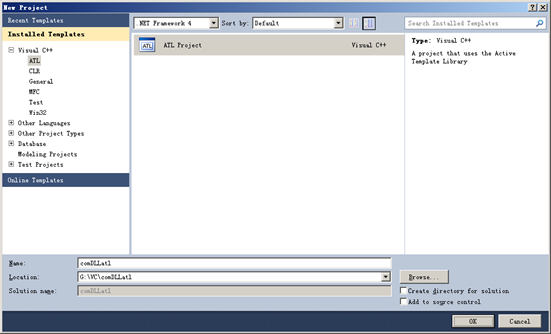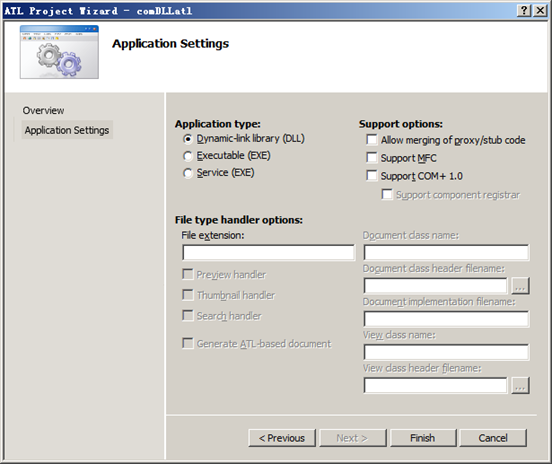1.3 增加COM

1.3.1 VC++6.0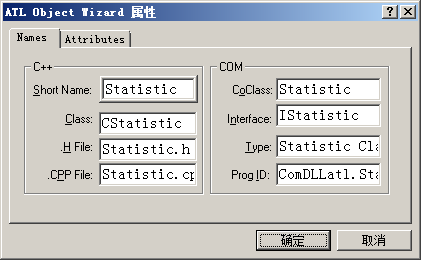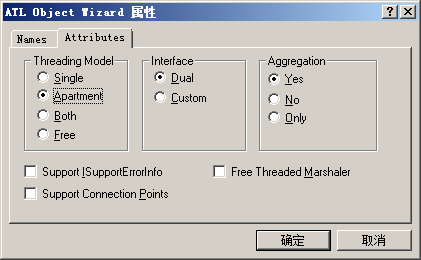1.3.2 VC++2010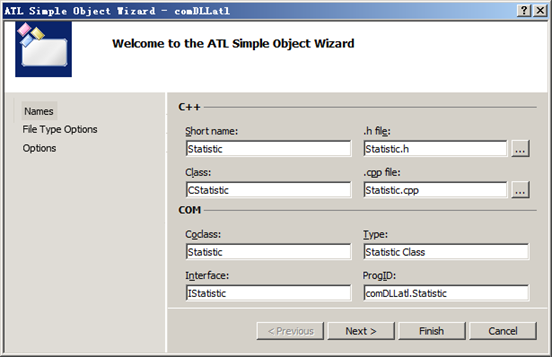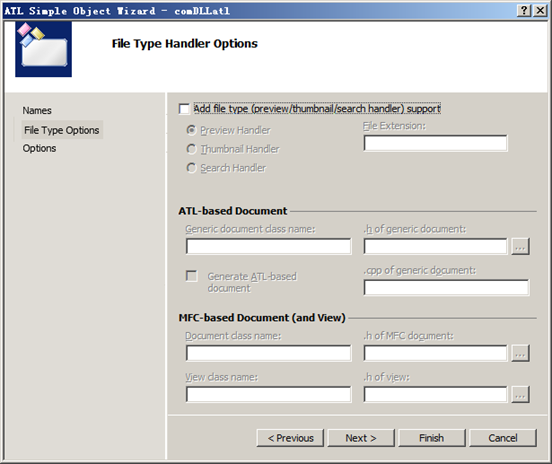1.3.3 项目结构

VC++6.0的类视图里增加了"CStatistic"和"IStatistic"。

IStatisticCOM接口，客户端程序通过它访问COM组件。

CStatisticCOM类，真正的工作由它来完成。// comDLLatl.idl import "oaidl.idl"; import "ocidl.idl"; [     uuid(0FEBC618-38BC-4A5B-AE09-3C56635F4D73),     version(1.0),     helpstring("comDLLatl 1.0 Type Library") ] library COMDLLATLLib {     importlib("stdole32.tlb");     importlib("stdole2.tlb");     [         object,         uuid(7FB59FA6-E715-4C23-9520-DE93293E0B5F),             helpstring("IStatistic Interface"),         pointer_default(unique)     ]     interface IStatistic : IUnknown     {     };     [         uuid(471BF24D-B793-44A1-9205-7BF36A6EB698),         helpstring("Statistic Class")     ]     coclass Statistic     {         [default] interface IStatistic;     }; };

1.4 增加方法

1.4.1 VC++6.0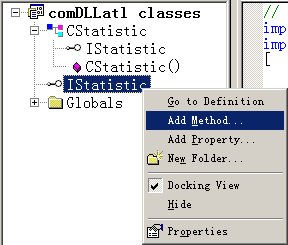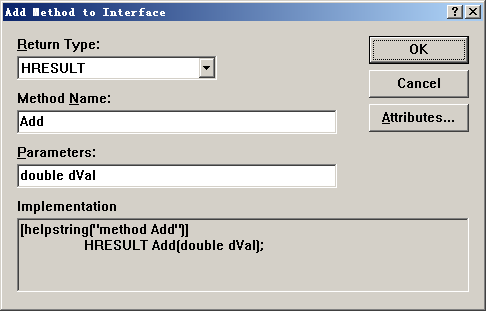1.4.2 VC++2010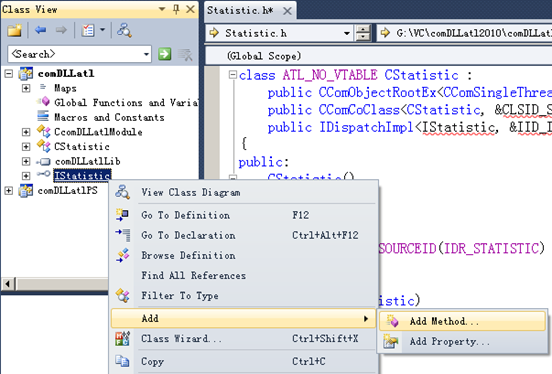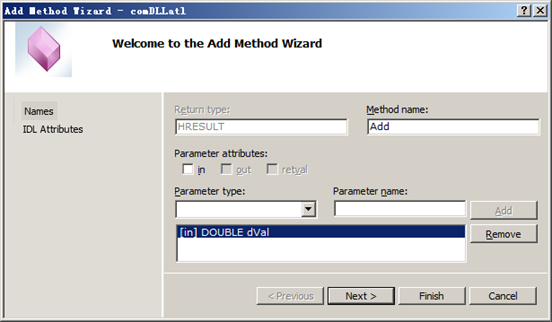1.5 增加属性

1.5.1 VC++6.0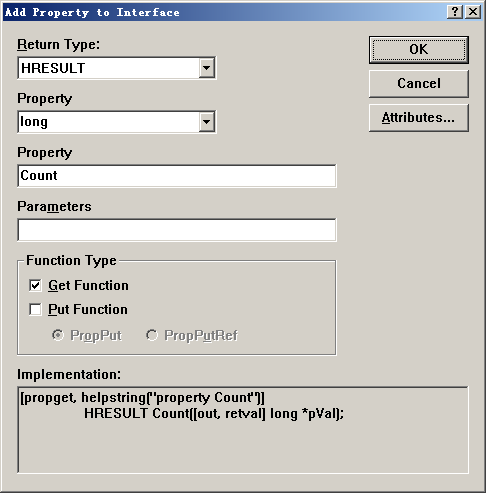1.5.2 VC++2010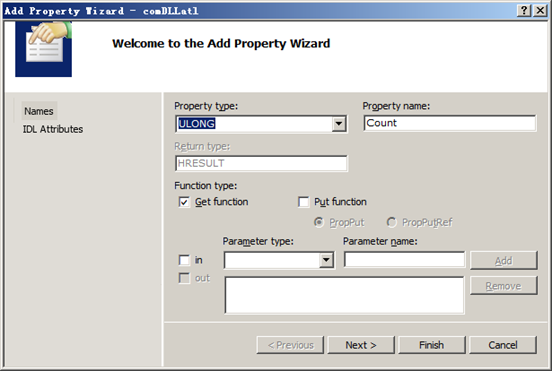1.6 删除方法、属性

1.7 编码

1.7.1 增加成员变量

 private: ULONG    m_nCount; double        m_dSum; double        m_dSum2;

1.7.2 初始化成员变量

CStatistic的构造函数里，初始化这三个成员变量

 CStatistic() { m_nCount        =    0; m_dSum        =    0.0; m_dSum2        =    0.0; }

 STDMETHODIMP CStatistic::Add(double dVal) { ++m_nCount;             //样本个数 m_dSum += dVal;         //所有样本值的和 m_dSum2 += dVal * dVal;     //所有样本值的平方和 return S_OK; }

1.7.4 实现Reset

 STDMETHODIMP CStatistic::Reset() { m_nCount = 0; m_dSum = 0.0; m_dSum2 = 0.0; return S_OK; }

1.7.5 实现get_Count

 STDMETHODIMP CStatistic::get_Count(long *pVal) { *pVal = m_nCount; return S_OK; }

1.7.6 实现get_Average

 STDMETHODIMP CStatistic::get_Average(double *pVal) { if(m_nCount) { *pVal = m_dSum / m_nCount; } else { *pVal = 0.0; } return S_OK; }

1.7.7 实现get_StdDev

 STDMETHODIMP CStatistic::get_StdDev(double *pVal) { *pVal = 0.0; if(m_nCount > 1) { *pVal = (m_nCount * m_dSum2 - m_dSum * m_dSum) / (m_nCount * (m_nCount - 1)); if(*pVal > 0.0) { *pVal = sqrt(*pVal); } } return S_OK; }

1.8 注册、注销

 regsvr32 comDLLatl.dll Rundll32 comDLLatl.dll,DllRegisterServer

 regsvr32 /u comDLLatl.dll Rundll32 comDLLatl.dll,DllUnregisterServer

 HKEY_LOCAL_MACHINE\SOFTWARE\Classes\TypeLib\ HKEY_LOCAL_MACHINE\SOFTWARE\Classes\Interface\ HKEY_LOCAL_MACHINE\SOFTWARE\Classes\CLSID HKEY_LOCAL_MACHINE\SOFTWARE\Classes\

2 VC++使用组件

2.1 #import

2.2 MFC包装类

2.3 C语言调用